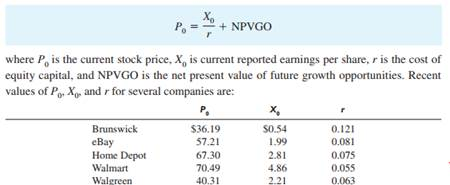### Create an Account

Home / Questions / As shown in equation 69 the price equation for a firm with positive growth opportunities i...

# As shown in equation 69 the price equation for a firm with positive growth opportunities is Required 1 Why does eBay have a higher cost of equity capital r than Wal Mart 2 Compute

As shown in equation (6.9), the price equation for a firm with positive growth opportunities isRequired:

1. Why does eBay have a higher cost of equity capital (r) than Wal-Mart?

2. Compute NPVGO for each company.

3. Compute NPVGO as a percent of stock price for each company.

4. Why is eBay’s NPVGO as a percent of stock price greater than Home Depot’s?

5. Why is Walmart’s NPVGO negative?

Required:

1. Why does eBay have a higher cost of equity capital (r) than Wal-Mart?

2. Compute NPVGO for each company.

3. Compute NPVGO as a percent of stock price for each company.

4. Why is eBay’s NPVGO as a percent of stock price greater than Home Depot’s?

5. Why is Walmart’s NPVGO negative?

May 25 2020 View more View LessSubscribe To Get Solution